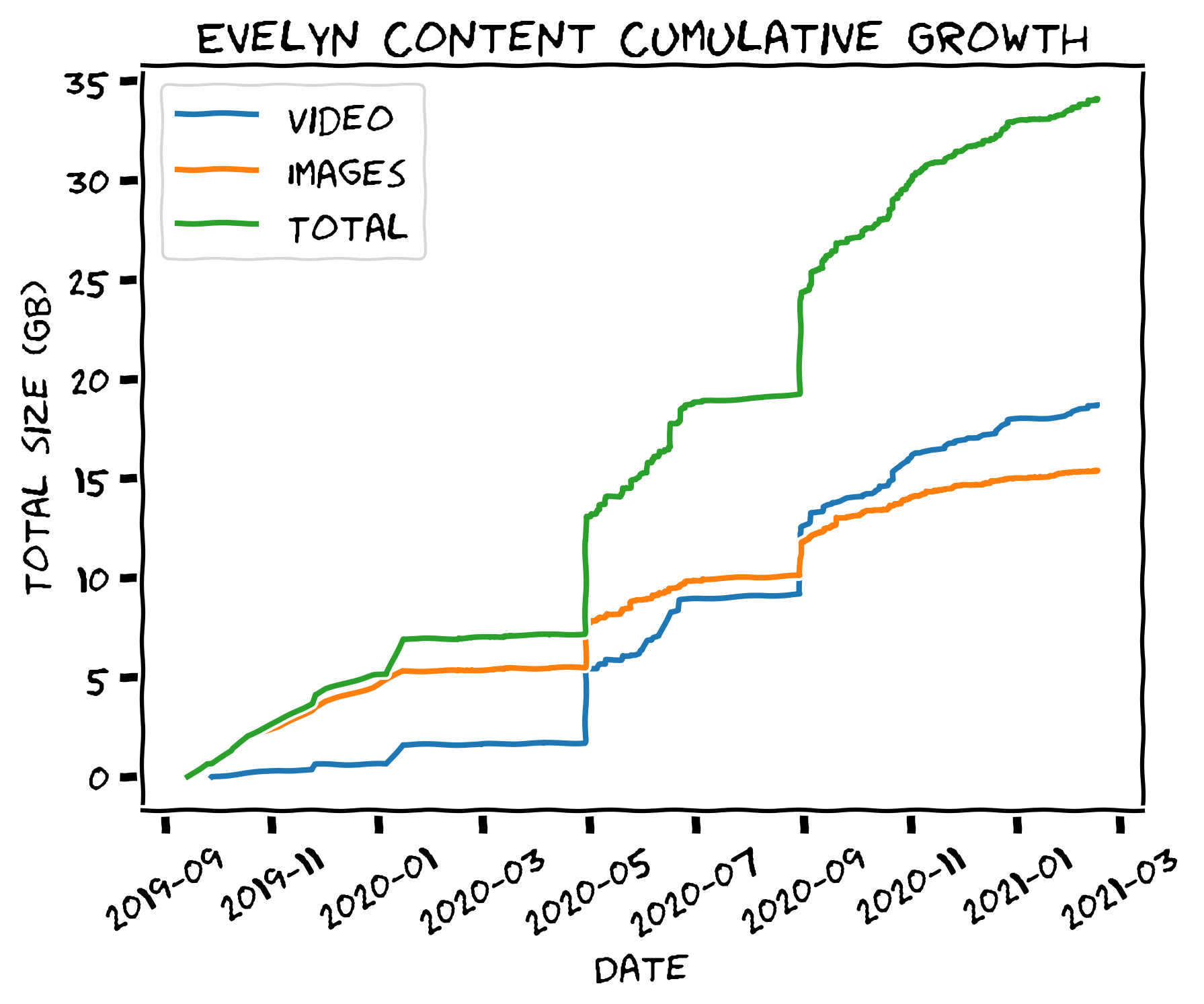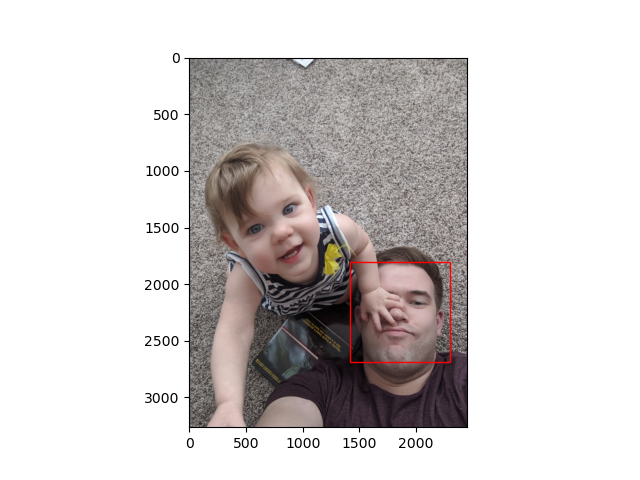One of the most common applications of machine learning is facial recognition. Plenty of examples of this exist, including Apple’s face unlock for iPhone, Windows Hello, and Google’s clustering of faces in the Google Photos app. I thought it would be fun to build something that could find my daughter’s face in family pictures, using just the data that I have. Incidentally, here is how much media I have with her in it:Should be easy, right? Well, doing it well and understanding it took a week or two (mostly in the evenings after work and grading student’s homework for the class I teach).

# Training Pipeline

The central idea is that I wanted to build an image processing pipeline. There are two parts to this: a training pipeline, and a classification pipeline.

## Generating image chips

Step one is collecting data for training the classifiers. This was pretty easy, as I have years of family pictures on my hard drive:

chris@dijkstra [12:58:56 PM] [~]
-> % du -mach ~/Pictures/family --max-depth=1 | grep total
44G	total
chris@dijkstra [12:59:04 PM] [~]
-> %


Plenty of images there to train on. The first piece of code is an image chipper. Because the important part of an image is where the face is, we want to get labeled data where the faces are. This is done by chipping the image, extracting a subset of the image where interesting features are. For this I used scikit-learn’s Haar Cascade detector to find regions likely to contain a face. Cascade classifiers aren’t intuitively designed for facial recognition (you could use cascade classification to find cars, for example), so part of setting up the detector is choosing the configuration:

In : import magic
...: import skimage.io as skio
...: from skimage import data
...: from skimage.color import gray2rgb, rgba2rgb

In : %pylab
Using matplotlib backend: Qt5Agg
Populating the interactive namespace from numpy and matplotlib

In :


For demonstration purposes, we’ll use a picture of me and Evelyn, my daughter:

In : plt.imshow(img)
Out: <matplotlib.image.AxesImage at 0x7f1ca67ec4c0>

In :For the cascade detector, the user has to define the scale, step ratio, and min/max sizes. A variety of cameras and image resolutions are present in the dataset, so we’ll have a fairly large sweep range:

In : detections = detector.detect_multi_scale(
...:     img,
...:     scale_factor=1.1,
...:     step_ratio=2.0,
...:     min_size=(100, 100),
...:     max_size=(2000, 2000),
...: )

In : detections
Out: [{'r': 1887, 'c': 1442, 'width': 854, 'height': 854}]

In :


Detections are returned as the location of the upper left pixel (r is a row, and c is a column), the width, and the height. Plotting the single detection (later on we’ll discuss why it didn’t detect both faces) shows that the detector found my face pretty well:

In : detection = detections

In : x = detection["c"]
...: y = detection["r"]
...: height = detection["height"]
...: width = detection["width"]

patches.Rectangle((x, y), width, height, edgecolor="red", facecolor="none")
)
Out: <matplotlib.patches.Rectangle at 0x7ff544597d00>

In :This is enough to get us a good dataset. For this I wrote a program called chipper.py, which recursively searches an image directory and chips faces out of all of the images it finds. The main loop looks like this:

for path in tqdm(image_paths):
# We're skipping already chipped images
if list(args.output_dir.glob(f"{path.name}.chip.*.png")):
continue

# If something goes wrong, just toss that image out and continue
try:
except SyntaxError:
print(f"SyntaxError on {path}")
continue
except OSError:
print(f"OSError on {path}")
continue
except ValueError:
print(f"ValueError on {path}")
continue

# Use RGB images for the face detector
if img.shape[-1] == 4:
img = rgba2rgb(img)
elif img.shape[-1] == 2:
img = gray2rgb(img)

detections = detector.detect_multi_scale(
img,
scale_factor=1.1,
step_ratio=2.0,
min_size=(100, 100),
max_size=(2000, 2000),
)
for index, detection in enumerate(detections):
x = detection["c"]
y = detection["r"]
height = detection["height"]
width = detection["width"]
with warnings.catch_warnings():
warnings.filterwarnings("ignore", category=UserWarning)
skio.imsave(
args.output_dir / f"{path.name}.chip.{index}.png",
img[y : y + height, x : x + width],
)


Very simple, although searching through 40+ GB of images does take a little while.

## Building a dataset

Once the images have all been chipped, I labeled them by placing them into labeled folders corresponding to the person whose face was in the chip. I also created a not_a_face label, to ensure there would be non-face examples in the training set.

To combine images into a usable dataset, I decided to use HDF5 to create a portable dataset with lower memory requirements. An HDF5 file has random access to the on-disk data, so a pipeline that would otherwise have high memory requirements can load data as needed, instead of having to load everything into memory at once.

### Handling different chip sizes

Because HDF5 stores image data as multidimensional NumPy arrays, images with differing dimensions cannot be stored together. To fix this, I wrote a scaler program that takes as input the path to a chip directory, and scales the images found there to be the median size found in each labeled set. The median size was chosen to make the changes to each chip as small as possible, given that there would be a variety of chip sizes. Most of the chips will probably be roughly the same size, which will minimize how much each chip needs to change.

To make the scaling process more efficient, the multiprocessing library is used to allow the user to devote multiple cores to processing the images. The process function is very simple:

def process(images_dir, n_jobs):
log.info("Calculating median image dimensions")

img_paths = []
dims = []
pbar = tqdm()
for subdir in images_dir.iterdir():
for img_path in subdir.iterdir():
if "scaled" in str(img_path):
continue
img_paths.append(img_path)
pbar.update()

dims = np.array(dims)

median_height = int(np.median(dims[:, 0]))
median_width = int(np.median(dims[:, 1]))

log.info("Scaling images to width %d and height %d", median_width, median_height)

with Pool(processes=n_jobs) as pool:
log.debug("Initialized pool with %d processes", n_jobs)
func = partial(
transform_and_save_image, height=median_height, width=median_width
)
pool.map(func, tqdm(img_paths))


After scaling the images, the dataset can be built by the next program. The interesting part of this program is the make_dataset function, which walks the chip directory and creates a labeled dataset by using the name of each directory where images with the .scaled suffix are present (the output of the scaler program).

def make_dataset(images_path, output_dir):
""" Train and return classifier

Args:
images_path (Path): Path to all labeled examples
output_dir (Path): Path to output HDF5 files to
"""
# First we need to assemble the data for the classifier,
# which can be done by scooping up all the images and checking
# where they came from. Everything that came from the name_path
# directory is a positive example, everything else is negative
img_paths = []
labels = []
for subdir in images_path.iterdir():
for img_path in subdir.iterdir():
if ".scaled" in img_path.suffixes:
img_paths.append(img_path)
labels.append(str(subdir.name).encode("ascii", "ignore"))

max_label_length = len(max(labels, key=lambda x: len(x)))

chips = []

log.info("Creating HDF5 dataset, max label length %d", max_label_length)
pbar = tqdm(total=len(img_paths))
with h5py.File(output_dir / f"dataset.h5", "w") as h5_file:
h5_file.create_dataset("labels", (len(labels), 1), f"S{max_label_length}", labels)
images_dataset = h5_file.create_dataset(
"images",
(height, width, len(img_paths)),
dtype=np.uint8,
)
for idx, img_path in tqdm(enumerate(img_paths)):
pbar.update()


The HDF5 file can be accessed directly like a NumPy array, without having to be loaded entirely into memory. The interface provides random access, which means a classifier will be able to load any arbitrary image by itself, without loading everything at once. The shape of the images are determined by checking the first image loaded, since each of the chips has been scaled to the median size.

## Training classifiers

To facilitate the creation of classifiers for different faces, I created a program to train a model using a name and a labeled dataset. The meat of this program is in the train_classifier function:

def train_classifier(
name,
dataset_path,
output_dir,
model,
*,
n_jobs=1,
cv=3,
orientations=8,
ppc=(16, 16),
cpb=(1, 1),
kernels=["linear", "rbf"],
C=np.arange(1, 10.5, 0.5),
max_depth=np.arange(10, 100, 10),
criterion=["entropy", "gini"],
):
""" Train and return classifier

Args:
name (str): String name of positive example
dataset (Path): Path to all labeled dataset
output_dir (Path): Path to output model to
model (str): Model type to create
n_jobs (int, optional): Number of processes, defaults to 1
cv (int, optional): Number of cross-validation folds, defaults to 3
orientations (int, optional):
Number of orientations to compute feature histograms for, defaults to 8
ppc (tuple, optional): Pixel per cell, defaults to (16, 16)
cpb (tuple, optional): Cells per block, defaults to (1, 1)
kernels (list, optional): Kernels for training an SVM, defaults to [linear, rbf]
C (list, optional):
List of regularization parameter values for training an SVM, defaults to np.arange(1, 10.5, 0.5)

Returns:
clf (BaseEstimator): Classifier trained on images